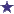# Ratio/Dilution Question: Order for 380ml of 0.5% dextrose and sterile water

by Anthony
(NY)38 mL Dextrose 5%

You receive an order for 380 ml of 0.5% dextrose solution. You have 1500 L of 5% dextrose solution, how much of the 5% solution and sterile water will you need to fill this order?

### Comments for Ratio/Dilution Question: Order for 380ml of 0.5% dextrose and sterile water

Average RatingRating38 ml of the 5% dextrose solution is needed by: David Thank you for the question, Anthony. To solve this question, we know that what the order calls for is a 0.5% dextrose solution. What we have is 5% dextrose solution. The 5% dextrose solution is a factor or 10 (or 10 times the 0.5%). So the total amount needed is 380mL. What we need is 10% of the 380mL ( =38mL ) of the 5% dextrose solution. Subtract 38mL from 380mL and you come up with 342mL. The 38mL of the 10X dextrose solution (5%) is diluted by the remaining 342mL, which makes a final product of 380mL of a 0.5% dextrose solution.38 mL 5% dextrose solution and 342 mL sterile water.